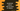# Example of JavaScript reduce with an array of objects## Example of JavaScript reduce with an array of objects:

JavaScript reduce is used to get a single value from an array. It takes one function and performs it on each element of the array from left to right. The result is stored in a different variable called accumulator.

reduce doesn’t change the original array, but it returns one single value by applying the function on each of its elements.

reduce can also be used with an array of objects.

In this post, I will show you how to use reduce with an array of objects in JavaScript.

### JavaScript example program:

Let’s take a look at the below example:

``````const givenArray = [
{ data: 1 },
{ data: 2 },
{ data: 3 },
{ data: 4 },
{ data: 5 },
];

const sum = givenArray.reduce((acc, curr) => acc + curr.data, 0);

console.log(sum);``````

Here,

• givenArray is the array of objects.
• This program is finding the sum of all data of the objects.
• reduce takes two parameters. The first one is a function that is used in all values of the array. The acc value is the accumulator and curr is the current object. It is accumulating the values of data of each object. The initial value of acc is 0.

It prints the below output:

``15``

This is the sum of all data of the objects.